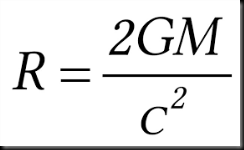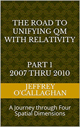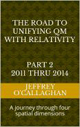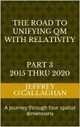# The math errors in the Big Bang TheoryThe Big Bang Theory is the leading explanation about how the universe began. At its simplest, it says the universe as we know it started with a small singularity, then inflated over the next 13.8 billion years to the cosmos that we know today.Because current instruments don’t allow astronomers to peer back at the universe’s birth, much of what we understand about the Big Bang Theory comes from mathematical formulas and models. Astronomers can, however, see the "echo" of the expansion through a phenomenon known as the cosmic microwave background.

The idea the universe was smaller in the beginning was supported by Edwin Hubble in 1929 it expanding.

Later, a few physicists led by George Gamow a proponent of the big bang model showed an expanding universe meant that it might have had its beginning in a very hot infinitely dense environment, which then expanded to generate the one we live in today.

They were able to show only radiation emitted approximately 300,000 years after the beginnings of the expansion should be visible today because before that time the universe was so hot that protons and electrons existed only as free ions making the universe opaque to radiation. This period is referred as the age of "recombination".

Additionally, they predicted this Cosmic Background Radiation or what was left over from the age of recombination would have cooled form several thousand degrees Kelvin back when it was generated to 2.7 today due to the expansion of the universe.  Many thought its discovery 1965 by Penzias and Wilson provided its verification

However, there was a problem with assuming the universe begin as an expansion of in an infinitely dense hot environment because one would expect it and the Cosmic Background Radiation to be homogeneous because an infinitely dense environment must have been, by definition homogeneous. Therefore, if the universe was homogeneous when it began it should still be.

But the existence of galactic clusters and the variations in the intensity of the cosmic background radiation discovered by NASA’s WMAP satellite showed the universe is not and therefore, was not homogeneous either now or at the time when the Cosmic Background Radiation was emitted.

Many proponents of the big bang model assume that these "anisotropy" in the universe are caused by quantum fluctuations in the energy density of space. They define quantum fluctuations as a temporary change in the energy of space caused by the uncertainty principle.

However, there is an error in the math used to predict both effects the expansion of singularity at its origin and quantum fluctuations in the energy density of space would have on the evolution of the universe.

Einstein mathematics tell us time slows as the gravitational or energy density increases and will eventually stop if it becomes great enough.  While observation of black holes provides verification of his math because it is observed that time does slow to a stop when it reaches a critical energy density at its event horizon.  Additionally, Schwarzschild was able to use Einstein’s math to calculate the radius of a black hole were the energy density would be great enough to stop time.

This means the math used by the proponents of the big bang is INCORRECT if they did not include the effect the energy density around a singularity or quantum fluctuation would have on its evolution.

This is because observationally verified math of Schwarzschild tells us there is a minimum radius the total energy content of the universe can occupy for time to move forward. Since evolution cannot occur in an environment where time has stopped that is also MINIMUM RADIUS of the universe which could expand form which IS larger than a singularity.

In other words, if they had included the effect energy density has on time, they would have realized that the universe could not have originated from a singularity.

However, there is a similar error in the math behind the assumption that quantum fluctuations are responsible for "anisotropy" in Cosmic Background Radiation because energy could not expand from one because the energy density surrounding it would cause time to stop.  Therefore, quantum fluctuation could not affect the evolution of the universe or be responsible for "anisotropy" in Cosmic Background Radiation because as was just mentioned evolution cannot occur in an environment where time has stopped.

Some might disagree because they say the energy in a singularity and that contained in a quantum fluctuation would be powerful enough to overcome the stopping of time predicted by Einstein mathematics.  However, they would be wrong because the mathematics of Einstein tells that when the energy density reaches a certain level time will stop.  It does not say that an increase beyond that point will allow time to move again.

As was mentioned earlier, current instruments don’t allow astronomers to peer back at the universe’s birth, much of what we understand about the Big Bang Theory comes from mathematical formulas and model

However, we may be able to define the origin of the present universe in terms of its observable properties.

We still have not been able to determine if the universe will continue to expand indefinitely or if it will eventually collapse in on itself. However, if one assumes it does, we could develop a mathematically model which would tell us when the heat generated by its collapse would be enough to cause it to re-expand.  Additionally, one could determine if that heat occurred AFTER that required to free protons and electrons from each other thereby allowing another age of "recombination" when it started to re-expand.

This would also give mathematicians the ability to more precisely determine the age of universe because we can observe when age of "recombination" occurred and project back from that point in time to when the additional heat generated by its continued collapse was great enough to cause it to re-expand.

In others words we have the ability to define the origin of the present universe and anisotropy" in Cosmic Background Radiation in terms of a mathematical model based on real time observations of the present universe.

The science of Astrophysics is base almost exclusively on observations.  Therefore, the question they must ask themselves is "If we have two models for the origin of the universe that predict the same outcome which one should we assume is correct?"  The one that make is predictions based on the observable properties of our present universe or one that defines it origins in terms of the unobservable properties of a singularity.

Copyright Jeffery B O’Callaghan Nov. 2020

The Road to unification part  2007 thru 2010  Ebook
 The Road to Unification part 1 2007 thru 2010Ebook \$8.00 Paper Back \$15.00 The Road to Unification part 2 2011 thru 2014Ebook \$8.00 Paper Back \$17.00 The Road to Unification part 3 2015 thru 2020Ebook \$8.00 Paper Back \$15.00

The post The math errors in the Big Bang Theory appeared first on Unifying Quantum and Relativistic Theories.

This post first appeared on Unification Of Quantum Mechanics With Relativity, please read the originial post: here